Miscellaneous

Miscellaneous

Including: Vectors, HYP Functions, Complex & Real Numbers.

Vectors

1) ABS
Calculates the norm of a vectror
The norm of a vector [ a b c ] is definite as SQRT(a^2 + b^2 + c^2)

Example::
Find the norm of the vector [2 3 4]
level 1: [2 3 4]
[MTH][VECTR][ABS]

Result: 5.38516480713

2) DOT
Calculates the scalar product of two vectors.
The result is a number.

Example::
[2 3 4].[6 7 8]
level 2: [2 3 4]
level 1: [6 7 8]
[MTH][VECTR][DOT]

Result: 65

3) CROSS
Calculates the vectorial produc of two vectors.
The result is a vector.

Example::
[2 3 4] x [6 7 8]
level 2: [2 3 4]
level 1:[6 7 8]
[MTH][VECTR][CROSS]

Result: [-4 8 -4]

4)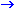V2
Convert numbers in levels 1 and 2 into a vector.

Example::
level 2: 9
level 1: 8

[MTH][VECTR][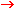V2]
Result: [9 8]

5)V3
Convert numbers in levels 1, 2 and 3 into a vector.

Example::
level 3: 4
level 2: 5
level 1: 8
[MTH][VECTR][V3]

Result: [4 5 8]

6) VConvert a vector into stack numbers.

Example::
level 1:[2 6 1 ]
[MTH][VECTR][V]

Result:
level 3: 2
level 2: 6
level 1: 1

Hyperbolic Functions

Hyperbolic functions are functions based on exp(x)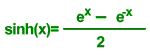To find the value of an hyperbolic trigonometric
funtion all you need is to put the number
on the stack and press the respective function at [MTH][HYP]

Example::
Calculate the sinh(5)

level 1: 5
[MTH][HYPER][SINH]

Result: 74.2032105778

Real & Complexes Numbers
In HP48/49 complexes numbers are represented between ( ).
as (real part, complex part)
Work with complexes numbers in HP48/49 is easy.
Below are listed the most useful functions.

1) RE
Returns the real part of a complex number

Example::
Find the real part of (123, 456)

Level 1: (123, 456)
Press [MTH][NXT][CMPL][RE]

Result: 123

2) IM
Returns the imaginary part of a complex number

Example::

Find the imaginary part of (123, 456)

Level 1: (123, 456)
Press [MTH][NXT][CMPL][IM]
Result: 456

3) ABS
Returns the norma of a complex number

Example::
Find the norm of the complex number (123, 456)

Level 1: (123, 456)
Press [MTH][NXT][CMPL][ABS]

Result: 472.297575687

4) ARG
Return the angle argument of a complex number

Example::
Find the angle argument of (123, 456)

Level 1: (123, 456)
Press [MTH][NXT][CMPL][ARG]

Result:
74.9044791558 (angle mode)

5) CONJ
Return the conjugate of a complex number

Example::
Find the conjugate of (123, 456)

Level 1: (123, 456)
Press [MTH][NXT][CMPL][ARG]
Result: (123, -456)

Real Numbers

1) RND
Rounds a number for a definite numbers of digits

Level 2: 1.8678987
Level 1:3
Press [MTH][REAL][RDN]

Result: 1.868

2) TRNC
Truncates a number to the set numbers of digits

Level 2: 1.8678987
Level 1:3
Press [MTH][REAL][TRNC]

Result: 1.867

3) MOD
Returns the rest of a division between two numbers

Example::

Level 2: 14
Level 1:3
Press [MTH][REAL][MOD]

Result: 2
Note: 8 / 3 = 4 and rest 2.

4) IP
Show the Integer Part of a number

Example::

Level 2: 1.8678987
Press [MTH][REAL][IP]

Result: 1

5) FP
Show the Fraction Part of a number

Example:

Level 2: 1.8678987
Press [MTH][REAL][FP]

Result:. 8678987

6) DR

Example:

Level 2: 45
Press [MTH][REAL][DR]

Result: .785398163397

7) RD

Example:

Level 2: .785398163397 Example:

Press [MTH][REAL][RD]

Result: 45

8) CEIL
Returns the mayor integer closest to the number

Example:

Level 2: 1,45
Press [MTH][REAL][CEIL]

Result: 2

9) FLOOR
Returns the minor integer closest to the number

Example:

Level 2: 1,45
Press [MTH][REAL][FLOOR]

Result: 1

10) %
Returns the percentage

Example:

Level 2: 100
Level 1: 12,67
Press [MTH][REAL][%]

Result:12.67

Eigenvectors and Eigenvalues
1) EGV
The command EGV calculates the eigenvalues and the eigenvectors of a given matrix.

Example:

Calculates the eigenvalues and the eigenvectors of the matrix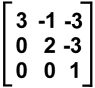Resolution:
1- write the matrix in the HP48/49 as below: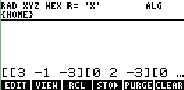and press [ENTER]

2- type EGV and press [ENTER]

It will show: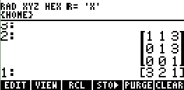where the columns of the matrix are the eigenvectors associated
to the eigenvalues shown in the vector below the matrix.

In this Example:,
[1 0 0] is the eigenvector associated to 3
[1 1 0] is the eigenvector associated to 2
[3 3 1] is the eigenvector associated to 1

2) EGVL
The command EGVL calculate the eigenvector of a given matrix

Example:

Calculates the eigenvalues and the eigenvectors of the matrixResolution:
1- write the matrix in the HP48/49 as below:and press [ENTER]

2- type EGVL and press [ENTER]

It shows the vector [ 3 2 1]
where 3, 2 and 1 are the eigenvalues for the matrix.

Note:
These Examples was done with HP49 mode set RPN

HP48 is also able to calculate eigenvalues and eigenvectors,
but HP48 shows the eigenvector matrix in line,
and eigenvalues as a vector.

For the Examples of EGV above it shows something like,
[ [ 1 1 3 ] [ 0 1 3 ] [ 0 0 1] ]
[ 3 2 1].

Press [SWAP] to see it like.

[ 3 2 1]
[ [ 1 1 3 ]
[ 0 1 3 ]
[ 0 0 1 ] ]

Where the eigenvectors are shown as colums of the matrix,
and the eigenvalues as the vector [ 3 2 1].

 If one has not given everything, one has given nothing. - Georges Guynemer